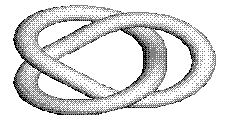# Knots ...Every one knows from experience how to create a knot. We do this all the time, often unwittingly. Knots whose ends were glued together and their classification form the subject of a branch of Topology known as the Knot Theory. On the left there is a picture of the Left Trefoil knot. On the right there is the Right Trefoil knot. It's impossible to continuously (i.e. stretching and twisting but without causing damage to either of them) deform one into another. However, it must be noted that the two knots are topologically equivalent in the sense that there exists a topological transformation that maps one into another. The two knots are mirror reflections of each other.

In the real world, it can be argued that mirror reflections are only mental images whose existence is entirely different from that of the objects whose reflections they are. In Mathematics, reflections are as real as the objects themselves. Mathematically, reflections are topological transformations that could not be carried out on the real world objects. But, as many mathematicians might remark, no mathematical transformation could possibly apply to a real world object in the first place.

### An aside

There is an unorthodox way to create a trefoil knot. Start as if creating a Moebius strip. But this time twist one end of the strip three half turns. Now cut the resulting (one-sided) strip along the middle line. You should get a trefoil knot. Other surfaces can be obtained by gluing and cutting a paper strip.For your amusement there is an avi movie that captures creation of a knot (437760 bytes).

It's an interesting activity in a Trigonometry or Analytic Geometry classes to figure out the set of equations that results in these pictures. I introduce an idea of what is involved on my Moebius strip page.

• Martin Gardner gives a beautiful introduction into the knot topology in his Knotted Doughnuts, W.H.Freeman and Co, 1986.
• Dr. Crypton gives a somewhat frivolous example of a topological transformation in his Dr. Crypton and His Problems.
• R. Courant and H. Robbins mention knots in What is Mathematics? In a chapter added to the 1996 edition, Ian Stuart expands their exposition with newer results.
• S. Barr, Experiments in Topology, Dover Publications, NY, 1989. The easiest yet most insightful introduction into Topology.Topology emerged as part of Geometry which did away with metric properties of shapes - angles and distances. For example, topologically, sphere and cube are one and the same object since one can be transformed continuously (i.e. with no cutting nor tearing) into another. Therefore, it's so much more remarkable that number invariants are still used to characterize topological objects.

In the Knot Theory until 1984 the main tool to tell the knots apart was the Alexander polynomials so named after the American mathematician J.W.Alexander. However, those did not distinguish between the two trefoil knots. For both knots the Alexander polynomial was t2-t+1. In 1984 a New Zealander Vaughan Jones working on some aspects of Mathematical Physics discovered (Jones) polynomials that later were generalized even further simultaneously and quite independently by five separate groups of mathematicians. Known as the HOMFLY (Hoste-Ocneanu-Millett-Freyd-Lickorish-Yetter), these polynomials in two variables give -2x2 - x4 + x2y2 for the left and -2x2 - x-4 + x-2y2 for the right trefoil knots, respectively.

I mention these elements of the Knot Theory for two reasons. One is that with all the specialization of tools and interests due to the growth of the body Mathematics, this science is unified in that the basic strains permeate virtually every branch of Mathematics and this is a regular occurrence to detect links between distinct mathematical theories.

The second reason is that there are numerous examples in the history of mathematics when an important discovery has been made simultaneously by independent researchers as if an idea was floating in, I do not know where - air, the World of Numbers or common consciousness - waiting to be grasped by human minds. The end to the philosophical disscusion whether mathematics is being invented or discovered is nowhere in sight.Knots are discussed on Internet:

• Knots on the Web. I'd have to forgo my job, my family and my hobbies to explore this page
• Knot Theory in the Geometry Junkyard - a compendium of math related links### Elements of Topology